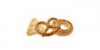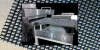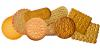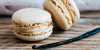Fat

What is the main difference  between slip melting point & SFC(Solid fat content) of Fat.

The SFC gives indeed the solids at a certain temperature. So SFC20=50 means 50% solids at 20°C as measured following AOCS Cd 16b-93

There are more ways to measure a melting point. The most common one is the Whiley melting point, measured as given in AOCS Method Cc 2-381. The slip melting point is usually the temperature where there stil about 3% solids. is. SFC values are more meaningful. Ftas with the same melting point can have e.g different SFC20 varying over a long range (35-75)

I think the slip melting point is expressed as the first temperature at which the fat starts to melt. SFC, on the other hand, expresses the amount of solid fats made at different temperatures.

The N-Value gives the percentage of fat which is solid or in the crystallised form at a given temperature:
N20= 35 indicates that 35% of the fat is in crystallised form at 20°C (the amount of solids at that temperature)
These values are measured with NMR-equipment (after heating to 60°C, stored for one hour at 0°C and half hour at measuring temperature).
These values are not always the same as the SFC-values (solid fats content) as used in the USA and measured on a different way.

Water is fully crystallising at 0°C (one type of molecule: H2O). But fat, consisting of a mixture of different triglycerides, has a melting or crystallising range. The lower the temperature the more triglycerides are crystallised and so the larger the part of the fat in the solid form.

N-line

The N-line is a line indicating the solids as function of temperature; usually a few N-values are used: N10, N20, N30 and N35. Examples are given in other pages.

Melting point

A few types of melting points are used in the literature:

• The Slip melting point: in practice the temperature where only a few percent of the fat is in the solids form. Practically this melting point can be derived from the N-line (the melting point is the temperature where 3-5% solids are present).
• The Wiley melting point, mainly used in the USA; gives a few degrees higher temperature than the slip melting point.
More detailed information can be obtained from standard handbooks on fats.
Help Tanmoy Basak by registering and answering.
Register now
Top TechTalkers

The score is based on the number of published articles (20 points for every approved article), number of accepted answers (10 points for every accepted answer) and the number of asked questions (3 points for every asked question). The list is showing only top 10 TechTalkers.Related articlesExplore other categories
Join the platform